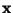Next: Projection pursuit Up: Classical Linear Transformations Previous: Factor analysis

## Higher-order methods

Higher-order methods use information on the distribution ofthat is not contained in the covariance matrix. In order for this to be meaningful, the distribution ofmust not be assumed to be Gaussian, because all the information of (zero-mean) Gaussian variables is contained in the covariance matrix. For more general families of density functions, however, the representation problem has more degrees of freedom. Thus much more sophisticated techniques may be constructed for non-Gaussian random variables.

Indeed, the transform defined by second-order methods like PCA is not useful for many purposes where optimal reduction of dimension in the mean-square sense is not needed. This is because PCA neglects such aspects of non-Gaussian data as clustering and independence of the components (which, for non-Gaussian data, is not the same as uncorrelatedness), as illustrated in Fig. 2. We shall here review three conventional methods based on higher-order statistics: projection pursuit, redundancy reduction, and blind deconvolution.

Aapo Hyvarinen
1999-04-23